# Vectors

### Term 2 starting in week 5 :: Estimated time: 2 weeks

• Understand and represent vectors
• Use and read vector notation
• Draw and understand vectors multiplied by a scalar
• Draw and understand addition of vectors
• Draw and understand addition and subtraction of vectors

For higher-attaining pupils:

• Explore vector journeys in shapes
• Understand parallel vectors
• Explore collinear points using vectors
• Use vectors to construct geometric arguments and proofs

This page should remember your ticks from one visit to the next for a period of time. It does this by using Local Storage so the information is saved only on the computer you are working on right now.

## Lesson Starters

Here are some suggestions for whole-class, projectable resources which can be used at the beginnings of each lesson in this block.

### 1st Lesson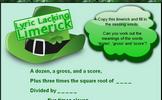#### Lyric Lacking Limerick

Find the words missing from the mathematical limerick.

### 2nd Lesson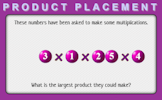#### Product Placement

Arrange the numbers to produce the largest product.

### 3rd Lesson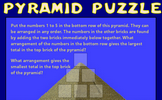#### Pyramid Puzzle

Arrange numbers at the bottom of the pyramid which will give the largest total at the top.

### 4th Lesson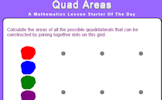Calculate the areas of all the possible quadrilaterals that can be constructed by joining together dots on this grid.

### 5th Lesson#### Roman Numerals

Learn a very different method for writing numbers using Roman numerals.

### 6th Lesson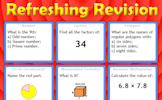#### Refreshing Revision

It is called Refreshing Revision because every time you refresh the page you get different revision questions.

Some of the Starters above are to reinforce concepts learnt, others are to introduce new ideas while others are on unrelated topics designed for retrieval practice or and opportunity to develop problem-solving skills.

White Rose ResourcesEnd of block assessments provide a quick progress check at the end of each block of learning to make sure students have understood the content covered. This Scheme of Learning was produced by White Rose Maths and is used here with permission granted on 30th June 2021.For All: